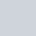in

# Data Types in R

In this article, we will study the various available data types in R and where they are used. Data types form the basic building blocks of any programming language. R is a dynamically typed language. Therefore, there is no need to make an explicit declaration of the data type of the variable. Each variable is associated with a data type on the basis of which memory allocation is done. For instance, the integer data type occupies 2 bytes and the character variable occupies 1 byte, respectively.

## Types of data types in R

R contains a large number of data types, some of which are namely:

### Logical data types in R

The logical data types in R are basically the boolean type R objects. There is assigned a boolean TRUE or FALSE value. The TRUE value can also be defined using the T value and FALSE using F respectively. Logical data types can also be assigned as a result of the condition evaluation.

```#declaring logical data type variables

#assigning True boolean value to var1
var1 = TRUE
#assigning False boolean value to var2
var2 = F
```

### Numeric data types in R

All sets of real numbers fall under the category of numeric data types. These may be decimals or whole numbers. The default data type for the numerical variables is numeric.

```#declaring numeric  data type variables

#example1
var1 = 3.42

#example2
var2 = 1/2

#example3
var3 = -5

#example 4
var4 = 9

#example5
var5 = 1e-3
class(var5)
```

### Integer data types in R

Integer data types are a subset of the numeric data type, which is inclusive only of numbers without decimal points. This implies that only integers are included in this category. Most such numbers are appended with the letter ‘L’. It may include negative or positive numbers.

```#declaring integer data type variables

#example1
var1 = 456L
#example2
var2 = -3L
#example3
var3 = 1/2L
```

Integer data types can also be declared using the method as.integer(num) which can convert any numeric data type to an integer.

```#declaring integer data type variables
#example1
var1 = 456L
#example2
var2 = -3L
#example3
var3 = 1/2L```

### Complex number in R

A complex number in mathematical form comprises a real number and an imaginary counterpart. In R, any variable is implicitly cast to the type of complex number if it is appended with the letter ‘i’.

```#declaring complex number data type variables
#example1
var1 = i

#example2
var2 = 2-2i
```

### String data type in R

The string or character data types comprise the letters, numbers, or special characters. Any value enclosed within a single or double quotation mark is considered to belong to the string data type. It may contain dates as well.

```#declaring string variables
var1 = "FoxInfoTech"

var2 = '@'

var3 = "1970-03-12"
```

## Examining data types in R

There are a large number of functions in-built in R in order to understand the particular data type associated with any R object.

### class() method

The class() method is used to validate the data type associated with any variable. This method takes as a parameter the variable to be checked. It is the high-level denotion of the object.

```#declaring examples
var1 = i
var2 = 43
var3 = as.integer(var2)
var4 = "FoxInfoTech"
var5 = T
var6 = 1e-3
var7 = '%'
#checking the data type
cat("var1 : ",class(var1))
cat("var2 : ",class(var2))
cat("var3 : ",class(var3))
cat("var4 : ",class(var4))
cat("var5 : ",class(var5))
cat("var6 : ",class(var6))
cat("var7 : ",class(var7))
```

The code produces the following output :

```var1 :  integer
var2 :  numeric
var3 :  integer
var4 :  character
var5 :  logical
var6 :  numeric
var7 :  character
```

### typeof() method

The typeof() method also depicts which category the particular data type falls into. It is the low-level denotion of the object. For instance, the numeric data type is implicitly stored in the form of “double” value with two decimal points in it, that is, 5 is stored as 5.00 in R.

```#declaring examples
var1 = i
var2 = 43
var3 = as.integer(var2)
var4 = "FoxInfoTech"
var5 = T
var6 = 1e-3
var7 = '%'

cat("var1 : ",typeof(var1))
cat("var2 : ",typeof(var2))
cat("var3 : ",typeof(var3))
cat("var4 : ",typeof(var4))
cat("var5 : ",typeof(var5))
cat("var6 : ",typeof(var6))
cat("var7 : ",typeof(var7))
```

The code produces the following output :

```var1 :  integer
var2 :  double
var3 :  integer
var4 :  character
var5 :  logica
var6 :  double
var7 :  character```

### as.data_type() method

This method is used to simulate the conversion of a variable from one data type to another in R. The data_type that is specified in this method is the corresponding data type to which the value is mapped.

```#conversion of data type

var1= 5
cat("Initial var1 : ", class(var1))

#conversion to string
var2 = as.character(5)
cat("Final var2 : ", class(var2))```

The output produced is as follows :

```Initial var1 :  numeric
Final var2 :  character
```

Similarly, methods like as.logical or as.complex can also be used to carry out successive conversions.

## Written by Mallika Gupta

A software developer with a keen desire to explain in detail and with precision, every topic that I introduce to the user, be it in my content or my teaching. Enthusiastic about learning and sharing it.